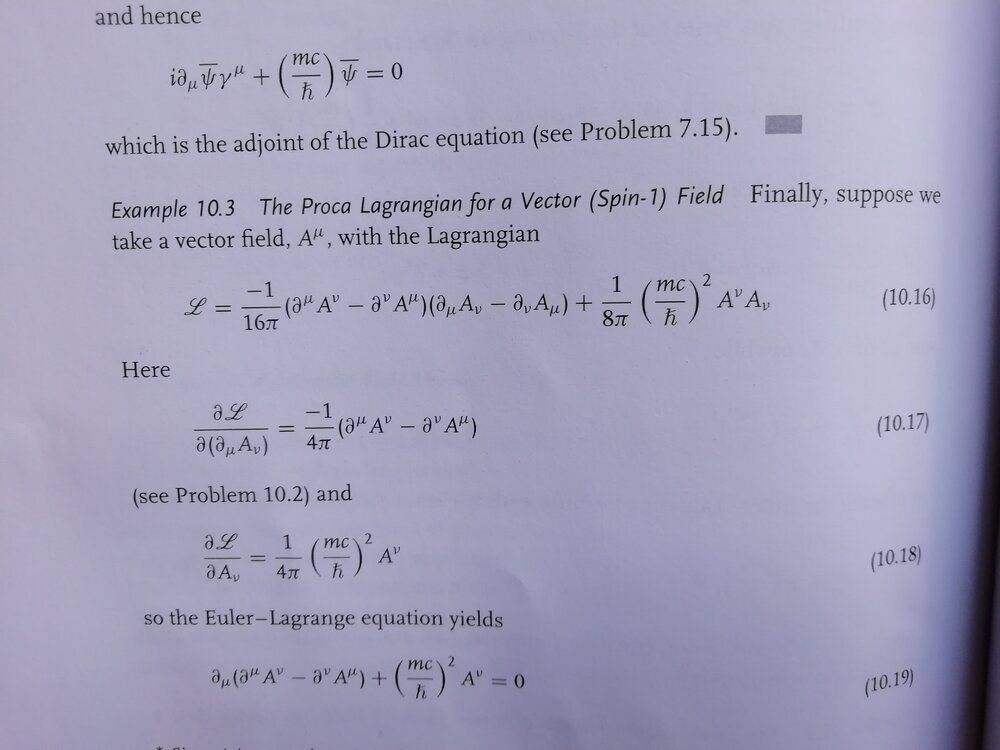# Simplification of the Proca Lagrangian

• fabstr1

#### fabstr1

Homework Statement
How do I simplify the Proca lagrangian for a spin-1 field
Relevant Equations
L = -(1/16*pi) * ( ∂^(μ)A^(ν) - ∂^(ν)A^(μ))(∂_(μ)A_(ν) - ∂_(ν)A_(μ)) + 1/(8*pi) * (mc/hbar)^2* A^ν A_ν
Hello,
I'm trying to figure out where the term (3) came from. This is from a textbook which doesn't explain how they do it.

∂_μ(∂L/(∂(∂_μA_ν)) = ∂L/∂A_ν (1)

L = -(1/16*pi) * ( ∂^(μ)A^(ν) - ∂^(ν)A^(μ))(∂_(μ)A_(ν) - ∂_(ν)A_(μ)) + 1/(8*pi) * (mc/hbar)^2* A^ν A_ν (2)

Here is Eq (1) the Euler-Lagrange equation and Eq (2) is the lagrangian for a vector field. In the textbook they just state the term

∂_μ(∂L/(∂(∂_μA_ν)) = -1/(4*pi)*(∂^(μ)A^(ν) - ∂^(ν)A^(μ)) (3)

Where does the term -1/(4*pi) come from, and how do I cancel out the rest of the term so that the equation becomes

∂_μ(∂^(μ)A^(ν) - ∂^(ν)A^(μ)) + (mc/hbar)^2* A^ν (4)•JD_PM

Hints:

##\mathcal{L}= -\frac{1}{16\pi}F^{\mu\nu}F_{\mu\nu} ##

Now:
##F^{\mu\nu}= \eta^{\mu n}\eta^{\nu m}F_{nm}##

Now use this in above equation and:

##F_{nm}=\partial_n A_m- \partial_m A_n##
And
##F_{nm}= -F_{mn}##

•JD_PM
But what happends to the rest of the term in Eq (10.17), where is the -1/(4*pi) term coming from.

L = - (1/(16*pi)) * η^(μν)η^(νμ)*F_(μν)

Last edited:
But what happends to the rest of the term in Eq (10.17), where is the -1/(4*pi) term coming from.

L = - (1/(16*pi)) * η^(μν)η^(νμ)*F_(μν)

Ok. I will do one more step for you.

##\mathcal{L}= \frac{-1}{16\pi} \eta^{\mu n}\eta^{\nu m} F_{nm}F_{\mu\nu}##

Now, taking the differential and applying product rule to above term throws a factor of 2(Due to symmetry of product) so this becomes (Dropping the metric tensors for brevity):

##\partial\mathcal{L}= \frac{-1}{8\pi} F_{nm}\partial F_{\mu\nu}##

Now, use the defination of ##F_{nm}##

I haven't taken any tensor calculus before, so I'm not sure if I'm doing it right.

∂L/∂F_{ μν } = - 1/(16*pi) * η^(μν)η^(νμ)*F_(μν)* F_(μν) = - 1/(16*pi)*η^(μν)η^(νμ)* F_(μν)^2 = -1/(8*pi)*η^(μν)η^(νμ)* F_(μν) = - 1/(8*pi)*η^(μν)η^(νμ)*(∂^(μ)A^(ν) - ∂^(ν)A^(μ))

I have updated my solution shown below. Is it ok, or is there something that are missing ?